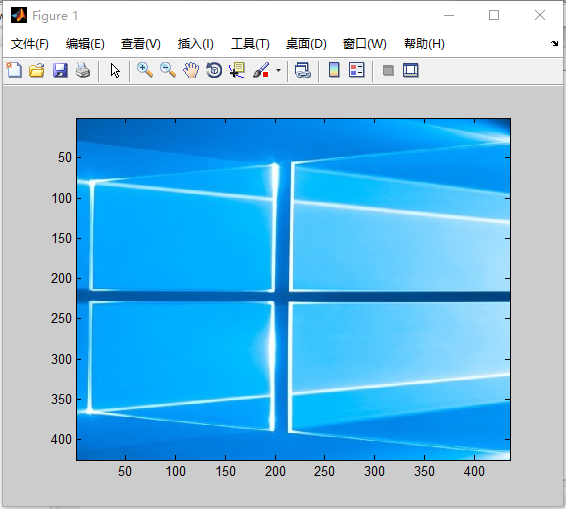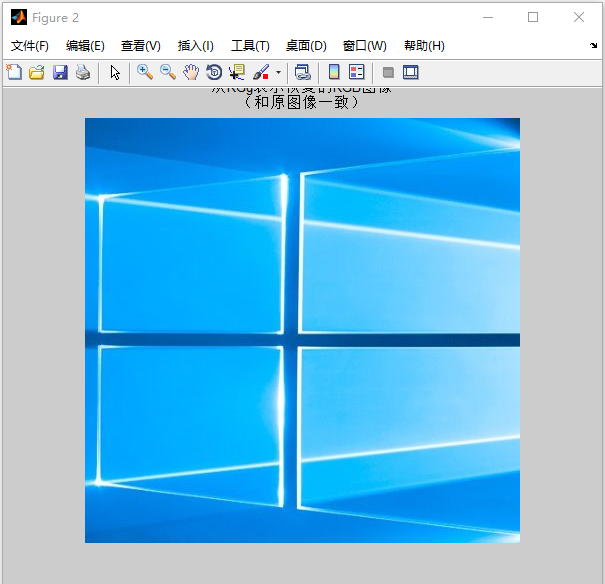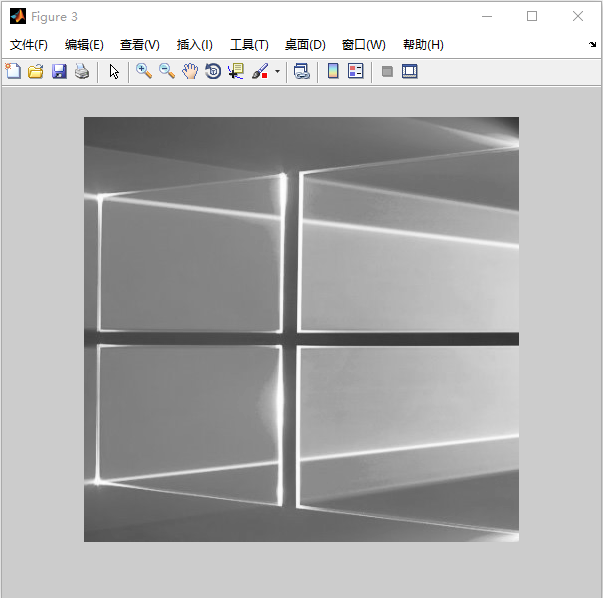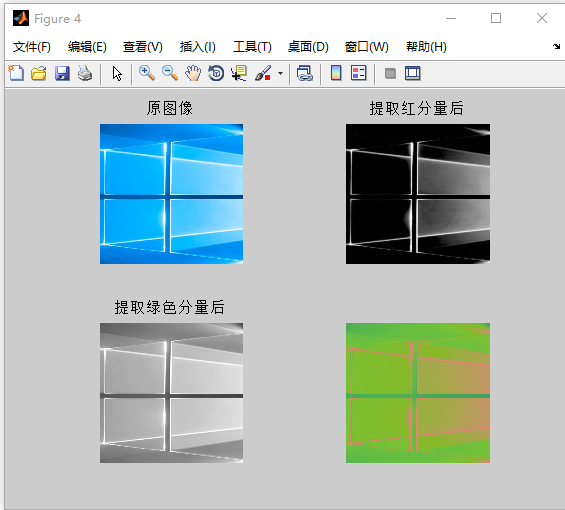• MATLAB图像处理常用测试图像 lena、Yacht、boat等
• ## matlab图像处理常用函数大全

万次阅读 多人点赞 2018-07-16 16:03:34
显示索引图像和灰度图像>> [X,map]=imread('trees.tif');>> gmap=rgb2gray(map);>> figure,imshow(X,map);>> figure,imshow(X,gmap);利用膨胀函数平移图像I = imread('football.jpg');se = translate(strel(1), [30 30]);%将一个平面结构化元素分别向下和向右移动30个位置J = imdilate(I,se);%利用膨胀函数平移图像subplot(121);imshow(I), title('原图')subplot(122), imshow(J), title('移动后的图像');水平翻转和上下翻转I = imread('cameraman.tif');Flip1=fliplr(I);               %  对矩阵I左右反转subplot(131);imshow(I);title('原图');subplot(132);imshow(Flip1);title('水平镜像');Flip2=flipud(I);               %  对矩阵I垂直反转subplot(133);imshow(Flip2);title('竖直镜像');图像旋转I=imread('cameraman.tif');B=imrotate(I,60,'bilinear','crop');%双线性插值法旋转图像，并裁剪图像，使其和原图像大小一致subplot(121),imshow(I),title('原图');subplot(122),imshow(B),title('旋转图像60^{o}，并剪切图像');截取图像I = imread('circuit.tif');I2 = imcrop(I,[75 68 130 112]);imshow(I), figure, imshow(I2)画轮廓>> I=imread('circuit.tif');>> imshow(I)>> figure>> imcontour(I,3)噪声和滤波I=imread('cameraman.tif');J=imnoise(I,'salt & pepper',0.02);%添加椒盐噪声subplot(121),imshow(J);title('噪声图像')K=medfilt2(J);%使用3*3的邻域窗的中值滤波subplot(122),imshow(K);title('中值滤波后图像')Matlab数字数字图像处理函数汇总：1、数字数字图像的变换① fft2：fft2函数用于数字数字图像的二维傅立叶变换，如：i=imread('104_8.tif');j=fft2(i);②ifft2:：ifft2函数用于数字数字图像的二维傅立叶反变换，如： i=imread('104_8.tif'); j=fft2(i);k=ifft2(j);2、模拟噪声生成函数和预定义滤波器① imnoise：用于对数字数字图像生成模拟噪声，如： i=imread('104_8.tif'); j=imnoise(i,'gaussian',0,0.02);%模拟高斯噪声② fspecial：用于产生预定义滤波器，如：h=fspecial('sobel');%sobel水平边缘增强滤波器h=fspecial('gaussian');%高斯低通滤波器h=fspecial('laplacian');%拉普拉斯滤波器h=fspecial('log');%高斯拉普拉斯（LoG）滤波器h=fspecial('average');%均值滤波器2、数字数字图像的增强①直方图：imhist函数用于数字数字图像的直方图显示，如：i=imread('104_8.tif');imhist(i);②直方图均化：histeq函数用于数字数字图像的直方图均化，如：i=imread('104_8.tif');j=histeq(i);imshow(J)③对比度调整：imadjust函数用于数字数字图像的对比度调整，如：i=imread('104_8.tif');j=imadjust(i,[0.3,0.7],[]);④对数变换：log函数用于数字数字图像的对数变换，如：i=imread('104_8.tif');j=double(i);k=log(j);⑤基于卷积的数字数字图像滤波函数：filter2函数用于数字数字图像滤波，如：i=imread('104_8.tif');h=[1,2,1;0,0,0;-1,-2,-1];j=filter2(h,i);⑥线性滤波：利用二维卷积conv2滤波, 如:i=imread('104_8.tif');h=[1,1,1;1,1,1;1,1,1];h=h/9;j=conv2(i,h);⑦中值滤波：medfilt2函数用于数字数字图像的中值滤波，如：i=imread('104_8.tif');j=medfilt2(i);⑧锐化（1）利用Sobel算子锐化数字数字图像, 如:i=imread('104_8.tif');h=[1,2,1;0,0,0;-1,-2,-1];%Sobel算子j=filter2(h,i);（2）利用拉氏算子锐化数字数字图像, 如:i=imread('104_8.tif');j=double(i);h=[0,1,0;1,-4,0;0,1,0];%拉氏算子k=conv2(j,h,'same');m=j-k;3、数字数字图像边缘检测①sobel算子 如：i=imread('104_8.tif');j =edge(i,'sobel',thresh) ②prewitt算子 如：i=imread('104_8.tif');j =edge(i,'prewitt',thresh)③roberts算子  如：i=imread('104_8.tif');j =edge(i,'roberts',thresh)④log算子  如：i=imread('104_8.tif');j =edge(i,'log',thresh)⑤canny算子 如：i=imread('104_8.tif');j =edge(i,'canny',thresh)⑥Zero-Cross算子 如：i=imread('104_8.tif');j =edge(i,'zerocross',thresh)4、形态学数字数字图像处理①膨胀：是在二值化数字数字图像中“加长”或“变粗”的操作，函数imdilate执行膨胀运算，如：a=imread('104_7.tif');%输入二值数字数字图像b=[0 1 0;1 1 1;01 0];c=imdilate(a,b);②腐蚀：函数imerode执行腐蚀，如：a=imread('104_7.tif');%输入二值数字数字图像b=strel('disk',1);c=imerode(a,b);③开运算：先腐蚀后膨胀称为开运算，用imopen来实现，如： a=imread('104_8.tif');b=strel('square',2);c=imopen(a,b);④闭运算：先膨胀后腐蚀称为闭运算，用imclose来实现，如： a=imread('104_8.tif');b=strel('square',2);c=imclose(a,b);  数字数字图像增强1. 直方图均衡化的 Matlab 实现1.1 imhist 函数功能：计算和显示数字数字图像的色彩直方图格式：imhist(I,n)        imhist(X,map)说明：imhist(I,n) 其中，n 为指定的灰度级数目，缺省值为256；imhist(X,map) 就算和显示索引色数字数字图像 X 的直方图，map为调色板。用stem(x,counts) 同样可以显示直方图。1.2 imcontour 函数功能：显示数字数字图像的等灰度值图格式：imcontour(I,n),imcontour(I,v)说明：n 为灰度级的个数，v 是有用户指定所选的等灰度级向量。1.3 imadjust 函数功能：通过直方图变换调整对比度格式：J=imadjust(I,[low high],[bottomtop],gamma)        newmap=imadjust(map,[low high],[bottomtop],gamma)说明：J=imadjust(I,[low high],[bottomtop],gamma) 其中，gamma 为校正量r，[lowhigh] 为原数字数字图像中要变换的灰度范围，[bottom top]指定了变换后的灰度范围；newmap=imadjust(map,[lowhigh],[bottom top],gamma) 调整索引色数字数字图像的调色板 map 。此时若 [low high] 和[bottom top] 都为2×3的矩阵，则分别调整 R、G、B 3个分量。1.4 histeq 函数功能：直方图均衡化格式：J=histeq(I,hgram)        J=histeq(I,n)        [J,T]=histeq(I,...)        newmap=histeq(X,map,hgram)        newmap=histeq(X,map)        [new,T]=histeq(X,...)说明：J=histeq(I,hgram) 实现了所谓“直方图规定化”，即将原是图象 I 的直方图变换成用户指定的向量 hgram 。hgram 中的每一个元素都在 [0,1] 中；J=histeq(I,n) 指定均衡化后的灰度级数 n ，缺省值为 64；[J,T]=histeq(I,...)返回从能将数字数字图像 I 的灰度直方图变换成数字数字图像 J 的直方图的变换 T ；newmap=histeq(X,map) 和 [new,T]=histeq(X,...) 是针对索引色数字数字图像调色板的直方图均衡。2. 噪声及其噪声的 Matlab 实现        imnoise 函数格式：J=imnoise(I,type)        J=imnoise(I,type,parameter)说明：J=imnoise(I,type) 返回对数字数字图像 I 添加典型噪声后的有噪数字数字图像 J ，参数type 和 parameter 用于确定噪声的类型和相应的参数。3. 数字数字图像滤波的 Matlab 实现3.1 conv2 函数功能：计算二维卷积格式：C=conv2(A,B)        C=conv2(Hcol,Hrow,A)        C=conv2(...,'shape')说明：对于 C=conv2(A,B) ，conv2 的算矩阵A 和 B 的卷积，若[Ma,Na]＝size(A), [Mb,Nb]=size(B), 则 size(C)=[Ma+Mb-1,Na+Nb-1];C=conv2(Hcol,Hrow,A) 中，矩阵 A 分别与Hcol 向量在列方向和 Hrow 向量在行方向上进行卷积；C=conv2(...,'shape') 用来指定 conv2返回二维卷积结果部分，参数 shape 可取值如下：        》full为缺省值，返回二维卷积的全部结果；        》same返回二维卷积结果中与 A 大小相同的中间部分；        valid 返回在卷积过程中，未使用边缘补 0 部分进行计算的卷积结果部分，当 size(A)>size(B) 时，size(C)=[Ma-Mb+1,Na-Nb+1]。3.2 conv 函数功能：计算多维卷积格式：与 conv2 函数相同3.3 filter2函数功能：计算二维线型数字滤波，它与函数 fspecial 连用格式：Y=filter2(B,X)        Y=filter2(B,X,'shape')说明：对于 Y=filter2(B,X) ，filter2 使用矩阵B 中的二维 FIR 滤波器对数据 X 进行滤波，结果 Y 是通过二维互相关计算出来的，其大小与 X 一样；对于Y=filter2(B,X,'shape') ，filter2返回的 Y 是通过二维互相关计算出来的，其大小由参数 shape 确定，其取值如下：        》full返回二维相关的全部结果，size(Y)>size(X)；        》same返回二维互相关结果的中间部分，Y 与X 大小相同；        》valid返回在二维互相关过程中，未使用边缘补 0 部分进行计算的结果部分，有 size(Y)<size(X) 。3.4 fspecial 函数功能：产生预定义滤波器格式：H=fspecial(type)        H=fspecial('gaussian',n,sigma)        高斯低通滤波器        H=fspecial('sobel')                        Sobel 水平边缘增强滤波器        H=fspecial('prewitt')                     Prewitt 水平边缘增强滤波器        H=fspecial('laplacian',alpha)            近似二维拉普拉斯运算滤波器        H=fspecial('log',n,sigma)                高斯拉普拉斯（LoG）运算滤波器        H=fspecial('average',n)                  均值滤波器        H=fspecial('unsharp',alpha)            模糊对比增强滤波器说明：对于形式 H=fspecial(type) ，fspecial 函数产生一个由 type 指定的二维滤波器 H ，返回的H 常与其它滤波器搭配使用。4. 彩色增强的 Matlab 实现4.1 imfilter函数功能：真彩色增强格式：B=imfilter(A,h)说明：将原始数字数字图像 A 按指定的滤波器 h 进行滤波增强处理，增强后的数字数字图像 B 与A 的尺寸和类型相同 数字数字图像的变换1. 离散傅立叶变换的Matlab 实现      Matlab 函数 fft、fft2 和 fftn 分别可以实现一维、二维和 N 维 DFT 算法；而函数 ifft、ifft2 和 ifftn 则用来计算反 DFT 。这些函数的调用格式如下：         A＝fft(X,N,DIM)      其中，X 表示输入数字数字图像；N 表示采样间隔点，如果 X 小于该数值，那么 Matlab 将会对 X 进行零填充，否则将进行截取，使之长度为N ；DIM 表示要进行离散傅立叶变换。        A＝fft2(X,MROWS,NCOLS) 其中，MROWS 和 NCOLS 指定对 X 进行零填充后的 X 大小。        A＝fftn(X,SIZE)其中，SIZE 是一个向量，它们每一个元素都将指定 X 相应维进行零填充后的长度。      函数 ifft、ifft2 和 ifftn的调用格式于对应的离散傅立叶变换函数一致。例子：数字数字图像的二维傅立叶频谱% 读入原始数字数字图像I＝imread('lena.bmp');imshow(I)% 求离散傅立叶频谱J=fftshift(fft2(I));figure;imshow(log(abs(J)),[8,10])2. 离散余弦变换的 Matlab实现2.1. dCT2 函数功能：二维 DCT 变换格式：B=dct2(A)         B=dct2(A,m,n)         B=dct2(A,[m,n]) 说明：B＝dct2(A) 计算 A 的 DCT 变换 B ，A 与 B 的大小相同；B＝dct2(A,m,n) 和 B=dct2(A,[m,n]) 通过对 A 补 0 或剪裁，使 B 的大小为 m×n。2.2. dict2 函数功能：DCT 反变换格式：B=idct2(A)         B=idct2(A,m,n)         B=idct2(A,[m,n]) 说明：B＝idct2(A) 计算 A 的 DCT 反变换 B ，A 与 B 的大小相同；B＝idct2(A,m,n) 和 B=idct2(A,[m,n]) 通过对 A 补 0 或剪裁，使 B的大小为 m×n。2.3. dctmtx函数功能：计算 DCT 变换矩阵格式：D＝dctmtx(n)说明：D＝dctmtx(n) 返回一个 n×n 的 DCT 变换矩阵，输出矩阵 D 为 double 类型。3. 数字数字图像小波变换的Matlab 实现3.1 一维小波变换的 Matlab实现(1) dwt 函数功能：一维离散小波变换格式：[cA,cD]=dwt(X,'wname')        [cA,cD]=dwt(X,Lo_D,Hi_D)说明：[cA,cD]=dwt(X,'wname')使用指定的小波基函数'wname' 对信号 X 进行分解，cA、cD分别为近似分量和细节分量；[cA,cD]=dwt(X,Lo_D,Hi_D) 使用指定的滤波器组 Lo_D、Hi_D 对信号进行分解。(2) idwt 函数功能：一维离散小波反变换格式：X=idwt(cA,cD,'wname')        X=idwt(cA,cD,Lo_R,Hi_R)        X=idwt(cA,cD,'wname',L)        X=idwt(cA,cD,Lo_R,Hi_R,L)说明：X=idwt(cA,cD,'wname')由近似分量 cA 和细节分量 cD 经小波反变换重构原始信号 X 。        'wname' 为所选的小波函数        X=idwt(cA,cD,Lo_R,Hi_R) 用指定的重构滤波器 Lo_R 和 Hi_R 经小波反变换重构原始信号 X 。        X=idwt(cA,cD,'wname',L) 和 X=idwt(cA,cD,Lo_R,Hi_R,L) 指定返回信号 X 中心附近的 L 个点。3.2 二维小波变换的 Matlab实现          二维小波变换的函数-------------------------------------------------     函数名                函数功能---------------------------------------------------     dwt2           二维离散小波变换   wavedec2       二维信号的多层小波分解     idwt2          二维离散小波反变换   waverec2        二维信号的多层小波重构   wrcoef2          由多层小波分解重构某一层的分解信号   upcoef2          由多层小波分解重构近似分量或细节分量   detcoef2         提取二维信号小波分解的细节分量   appcoef2        提取二维信号小波分解的近似分量   upwlev2         二维小波分解的单层重构   dwtpet2         二维周期小波变换   idwtper2        二维周期小波反变换-------------------------------------------------------------(1) wcodemat 函数功能：对数据矩阵进行伪彩色编码格式：Y=wcodemat(X,NB,OPT,ABSOL)        Y=wcodemat(X,NB,OPT)        Y=wcodemat(X,NB)        Y=wcodemat(X)说明：Y=wcodemat(X,NB,OPT,ABSOL)返回数据矩阵 X 的编码矩阵 Y ；NB 伪编码的最大值，即编码范围为 0～NB，缺省值 NB＝16；       OPT 指定了编码的方式（缺省值为 'mat'），即：                OPT＝'row' ，按行编码                OPT＝'col' ，按列编码                OPT＝'mat' ，按整个矩阵编码       ABSOL 是函数的控制参数（缺省值为 '1'），即：                ABSOL＝0 时，返回编码矩阵                ABSOL＝1 时，返回数据矩阵的绝对值ABS(X)(2) dwt2 函数功能：二维离散小波变换格式：[cA,cH,cV,cD]=dwt2(X,'wname')        [cA,cH,cV,cD]=dwt2(X,Lo_D,Hi_D)说明：[cA,cH,cV,cD]=dwt2(X,'wname')使用指定的小波基函数 'wname' 对二维信号 X 进行二维离散小波变幻；cA，cH,cV,cD 分别为近似分量、水平细节分量、垂直细节分量和对角细节分量；[cA,cH,cV,cD]=dwt2(X,Lo_D,Hi_D) 使用指定的分解低通和高通滤波器 Lo_D 和 Hi_D 分解信号 X 。(3) wavedec2 函数功能：二维信号的多层小波分解格式：[C,S]=wavedec2(X,N,'wname')        [C,S]=wavedec2(X,N,Lo_D,Hi_D)说明：[C,S]=wavedec2(X,N,'wname')使用小波基函数'wname' 对二维信号 X 进行 N 层分解；[C,S]=wavedec2(X,N,Lo_D,Hi_D) 使用指定的分解低通和高通滤波器Lo_D 和 Hi_D 分解信号 X 。(4) idwt2 函数功能：二维离散小波反变换格式：X=idwt2(cA,cH,cV,cD,'wname')        X=idwt2(cA,cH,cV,cD,Lo_R,Hi_R)        X=idwt2(cA,cH,cV,cD,'wname',S)        X=idwt2(cA,cH,cV,cD,Lo_R,Hi_R,S)说明：X=idwt2(cA,cH,cV,cD,'wname')由信号小波分解的近似信号cA 和细节信号 cH、cH、cV、cD 经小波反变换重构原信号 X；X=idwt2(cA,cH,cV,cD,Lo_R,Hi_R)使用指定的重构低通和高通滤波器 Lo_R 和 Hi_R 重构原信号 X ；X=idwt2(cA,cH,cV,cD,'wname',S)和X=idwt2(cA,cH,cV,cD,Lo_R,Hi_R,S) 返回中心附近的 S 个数据点。(5) waverec2 函数说明：二维信号的多层小波重构格式：X=waverec2(C,S,'wname')        X=waverec2(C,S,Lo_R,Hi_R)说明：X=waverec2(C,S,'wname')由多层二维小波分解的结果 C、S 重构原始信号 X ，'wname'为使用的小波基函数；X=waverec2(C,S,Lo_R,Hi_R) 使用重构低通和高通滤波器 Lo_R 和 Hi_R 重构原信号。  数字数字图像处理工具箱1. 数字数字图像和数字数字图像数据   缺省情况下，MATLAB将数字数字图像中的数据存储为双精度类型(double)，64位浮点数，所需存储量很大；MATLAB还支持另一种类型无符号整型(uint8)，即数字数字图像矩阵中每个数据占用1个字节。   在使用MATLAB工具箱时，一定要注意函数所要求的参数类型。另外，uint8与double两种类型数据的值域不同，编程需注意值域转换。          从uint8到double的转换   ---------------------------------------------       数字数字图像类型        MATLAB语句   ---------------------------------------------     索引色             B=double(A)+1     索引色或真彩色 B=double(A)/255     二值数字数字图像          B=double(A)   ---------------------------------------------         从double到uint8的转换   ---------------------------------------------       数字数字图像类型        MATLAB语句   ---------------------------------------------    索引色              B=uint8(round(A-1))    索引色或真彩色   B=uint8(round(A*255))    二值数字数字图像           B=logical(uint8(round(A)))   ---------------------------------------------2. 数字数字图像处理工具箱所支持的数字数字图像类型2.1 真彩色数字数字图像    R、G、B三个分量表示一个像素的颜色。如果要读取数字数字图像中(100,50)处的像素值，可查看三元数据(100,50,1:3)。    真彩色数字数字图像可用双精度存储，亮度值范围是[0,1]；比较符合习惯的存储方法是用无符号整型存储，亮度值范围[0,255]   2.2 索引色数字数字图像   包含两个结构，一个是调色板，另一个是数字数字图像数据矩阵。调色板是一个有3列和若干行的色彩映象矩阵，矩阵每行代表一种颜色，3列分别代表红、绿、蓝色强度的双精度数。      注意：MATLAB中调色板色彩强度[0,1]，0代表最暗，1代表最亮。          常用颜色的RGB值   --------------------------------------------    颜色   R   G   B      颜色    R  G   B    --------------------------------------------     黑     0   0   1     洋红    1   0   1     白     1   1   1     青蓝    0   1   1     红     1   0   0     天蓝 0.67 0   1     绿     0   1   0     橘黄    1 0.5 0     蓝     0   0   1     深红   0.5 0   0     黄     1   1   0      灰    0.5 0.5 0.5          --------------------------------------------         产生标准调色板的函数   -------------------------------------------------    函数名      调色板   -------------------------------------------------     Hsv       色彩饱和度，以红色开始，并以红色结束     Hot       黑色－红色－黄色－白色     Cool      青蓝和洋红的色度     Pink      粉红的色度     Gray      线型灰度     Bone      带蓝色的灰度     Jet        Hsv的一种变形，以蓝色开始，以蓝色结束     Copper    线型铜色度     Prim       三棱镜，交替为红、橘黄、黄、绿和天蓝     Flag       交替为红、白、蓝和黑--------------------------------------------------   缺省情况下，调用上述函数灰产生一个64×3的调色板，用户也可指定调色板大小。      索引色数字数字图像数据也有double和uint8两种类型。   当数字数字图像数据为double类型时，值1代表调色板中的第1行，值2代表第2行……   如果数字数字图像数据为uint8类型，0代表调色板的第一行，，值1代表第2行……2.3 灰度数字数字图像   存储灰度数字数字图像只需要一个数据矩阵。   数据类型可以是double，[0，1]；也可以是uint8，[0,255]2.4 二值数字数字图像   二值数字数字图像只需一个数据矩阵，每个像素只有两个灰度值，可以采用uint8或double类型存储。   MATLAB工具箱中以二值数字数字图像作为返回结果的函数都使用uint8类型。2.5 数字数字图像序列   MATLAB工具箱支持将多帧数字数字图像连接成数字数字图像序列。   数字数字图像序列是一个4维数组，数字数字图像帧的序号在数字数字图像的长、宽、颜色深度之后构成第4维。   分散的数字数字图像也可以合并成数字数字图像序列，前提是各数字数字图像尺寸必须相同，若是索引色数字数字图像，调色板也必须相同。   可参考cat()函数    A＝cat(4,A1,A2,A3,A4,A5)3. MATLAB数字数字图像类型转换         数字数字图像类型转换函数  ---------------------------------------------------------------------------     函数名                     函数功能  ---------------------------------------------------------------------------     dither       数字数字图像抖动，将灰度图变成二值图，或将真彩色数字数字图像抖动成索引色数字数字图像    gray2ind    将灰度数字数字图像转换成索引数字数字图像    grayslice    通过设定阈值将灰度数字数字图像转换成索引色数字数字图像     im2bw      通过设定亮度阈值将真彩色、索引色、灰度图转换成二值图    ind2gray    将索引色数字数字图像转换成灰度数字数字图像    ind2rgb      将索引色数字数字图像转换成真彩色数字数字图像    mat2gray   将一个数据矩阵转换成一副灰度图    rgb2gray    将一副真彩色数字数字图像转换成灰度数字数字图像    rgb2ind      将真彩色数字数字图像转换成索引色数字数字图像   ------------------------------------------------------------------------4. 数字数字图像文件的读写和查询4.1 图形数字数字图像文件的读取   利用函数imread()可完成图形数字数字图像文件的读取，语法：     A=imread(filename,fmt)     [X,map]=imread(filename,fmt)     [...]=imread(filename)     [...]=imread(filename,idx) （只对TIF格式的文件）     [...]=imread(filename,ref) （只对HDF格式的文件）   通常，读取的大多数数字图像均为8bit，当这些数字图像加载到内存中时，Matlab就将其存放在类uint8中。此为Matlab还支持16bit的PNG和TIF数字图像，当读取这类文件时，Matlab就将其存贮在uint16中。   注意：对于索引数字图像，即使数字图像阵列的本身为类uint8或类uint16，imread函数仍将颜色映象表读取并存贮到一个双精度的浮点类型的阵列中。4.2 图形数字图像文件的写入   使用imwrite函数，语法如下：   imwrite(A,filename,fmt)   imwrite(X,map,filename,fmt)   imwrite(...,filename)   imwrite(...,parameter,value)   当利用imwrite函数保存数字图像时，Matlab缺省的方式是将其简化道uint8的数据格式。4.3 图形数字图像文件信息的查询  imfinfo()函数5. 数字图像文件的显示5.1 索引数字图像及其显示   方法一：          image(X)          colormap(map)   方法二：           imshow(X,map)5.2 灰度数字图像及其显示   Matlab 7.0 中，要显示一副灰度数字图像，可以调用函数 imshow 或 imagesc （即 imagescale，数字图像缩放函数）   (1) imshow 函数显示灰度数字图像    使用imshow(I)    或 使用明确指定的灰度级书目：imshow(I,32)        由于Matlab自动对灰度数字图像进行标度以适合调色板的范围，因而可以使用自定义大小的调色板。其调用格式如下：           imshow(I,[low,high])    其中，low 和 high 分别为数据数组的最小值和最大值。   (2) imagesc 函数显示灰度数字图像   下面的代码是具有两个输入参数的 imagesc 函数显示一副灰度数字图像       imagesc(1,[0,1]);       colormap(gray);    imagesc 函数中的第二个参数确定灰度范围。灰度范围中的第一个值（通常是0），对应于颜色映象表中的第一个值（颜色），第二个值（通常是1）则对应与颜色映象表中的最后一个值（颜色）。灰度范围中间的值则线型对应与颜色映象表中剩余的值（颜色）。    在调用 imagesc 函数时，若只使用一个参数，可以用任意灰度范围显示数字图像。在该调用方式下，数据矩阵中的最小值对应于颜色映象表中的第一个颜色值，数据矩阵中的最大值对应于颜色映象表中的最后一个颜色值。5.3 RGB 数字图像及其显示   (1) image(RGB)    不管RGB数字图像的类型是double浮点型，还是 uint8 或 uint16 无符号整数型，Matlab都能通过 image 函数将其正确显示出来。   RGB8 = uint8(round(RGB64×255)); ％将 double 浮点型转换为 uint8 无符号整型   RGB64 = double(RGB8)/255;           ％ 将 uint8 无符号整型转换为 double 浮点型   RGB16 = uint16(round(RGB64×65535)); ％将 double 浮点型转换为 uint16 无符号整型    RGB64 = double(RGB16)/65535;      ％ 将 uint16 无符号整型转换为 double 浮点型   (2) imshow(RGB) 参数是一个 m×n×3 的数组5.4 二进制数字图像及其显示   (1) imshow(BW)   在 Matlab 7.0 中，二进制数字图像是一个逻辑类，仅包括 0 和 1 两个数值。像素 0 显示为黑色，像素 1 显示为白色。   显示时，也可通过NOT(~)命令，对二进制图象进行取反，使数值 0 显示为白色；1 显示为黑色。    例如：imshow(~BW)   (2) 此外，还可以使用一个调色板显示一副二进制数字图像。如果图形是 uint8 数据类型，则数值 0 显示为调色板的第一个颜色，数值 1 显示为第二个颜色。   例如：imshow(BW,[1 0 0;0 0 1])  5.5 直接从磁盘显示数字图像   可使用一下命令直接进行数字图像文件的显示：         imshow filename   其中，filename 为要显示的数字图像文件的文件名。   如果数字图像是多帧的，那么imshow 将仅显示第一帧。但需注意，在使用这种方式时，数字图像数据没有保存在Matlab7.0 工作平台。如果希望将数字图像装入工作台中，需使用 getimage 函数，从当前的句柄图形数字图像对象中获取数字图像数据，   命令形式为： rgb ＝ getimage;bwlabel 功能： 标注二进制数字图像中已连接的部分。 L = bwlabel(BW,n) [L,num] = bwlabel(BW,n)isbw 功能： 判断是否为二进制数字图像。 语法： flag = isbw(A) 相关命令： isind, isgray, isrgb 74．isgray 功能： 判断是否为灰度数字图像。 语法： flag = isgray(A) 相关命令： isbw, isind, isrgb11．bwselect 功能： 在二进制数字图像中选择对象。语法： BW2 = bwselect(BW1,c,r,n) BW2 = bwselect(BW1,n) [BW2,idx] = bwselect(...) 举例 BW1 = imread('text.tif'); c = [16 90 144]; r = [85 197 247]; BW2 = bwselect(BW1,c,r,4); imshow(BW1) figure, imshow(BW2)47．im2bw 功能： 转换数字图像为二进制数字图像。 语法： BW = im2bw(I,level) BW = im2bw(X,map,level) BW = im2bw(RGB,level) 举例 load trees BW = im2bw(X,map,0.4); imshow(X,map)
展开全文• 显示索引图像和灰度图像 >> [X,map]=imread('trees.tif'); >> gmap=rgb2gray(map); >> figure,imshow(X,map); >> figure,imshow(X,gmap); 利用膨胀函数平移图像 I = imread('football.jpg'); se = ...
显示索引图像和灰度图像
>> gmap=rgb2gray(map);
>> figure,imshow(X,map);
>> figure,imshow(X,gmap);
利用膨胀函数平移图像
se = translate(strel(1), [30 30]);%将一个平面结构化元素分别向下和向右移动30个位置
J = imdilate(I,se);%利用膨胀函数平移图像
subplot(121);imshow(I), title('原图')
subplot(122), imshow(J), title('移动后的图像');
水平翻转和上下翻转
Flip1=fliplr(I);               %  对矩阵I左右反转
subplot(131);imshow(I);title('原图');
subplot(132);imshow(Flip1);title('水平镜像');
Flip2=flipud(I);               %  对矩阵I垂直反转
subplot(133);imshow(Flip2);title('竖直镜像');
图像旋转
B=imrotate(I,60,'bilinear','crop');
%双线性插值法旋转图像，并裁剪图像，使其和原图像大小一致
subplot(121),imshow(I),title('原图');
subplot(122),imshow(B),title('旋转图像60^{o}，并剪切图像');
截取图像
I2 = imcrop(I,[75 68 130 112]);
imshow(I), figure, imshow(I2)
画轮廓
>> imshow(I)
>> figure
>> imcontour(I,3)
噪声和滤波
J=imnoise(I,'salt & pepper',0.02);%添加椒盐噪声
subplot(121),imshow(J);title('噪声图像')
K=medfilt2(J);%使用3*3的邻域窗的中值滤波
subplot(122),imshow(K);title('中值滤波后图像')
Matlab数字数字图像处理函数汇总：
1、数字数字图像的变换
j=fft2(i);
②ifft2:：ifft2函数用于数字数字图像的二维傅立叶反变换，如：
j=fft2(i);
k=ifft2(j);
2、模拟噪声生成函数和预定义滤波器
① imnoise：用于对数字数字图像生成模拟噪声，如：
j=imnoise(i,'gaussian',0,0.02);%模拟高斯噪声
② fspecial：用于产生预定义滤波器，如：
h=fspecial('sobel');%sobel水平边缘增强滤波器
h=fspecial('gaussian');%高斯低通滤波器
h=fspecial('laplacian');%拉普拉斯滤波器
h=fspecial('log');%高斯拉普拉斯（LoG）滤波器
h=fspecial('average');%均值滤波器
2、数字数字图像的增强
①直方图：imhist函数用于数字数字图像的直方图显示，如：
imhist(i);
②直方图均化：histeq函数用于数字数字图像的直方图均化，如：
j=histeq(i);
imshow(J)
④对数变换：log函数用于数字数字图像的对数变换，如：
j=double(i);
k=log(j);
h=[1,2,1;0,0,0;-1,-2,-1];
j=filter2(h,i);
⑥线性滤波：利用二维卷积conv2滤波, 如:
h=[1,1,1;1,1,1;1,1,1];
h=h/9;
j=conv2(i,h);
⑦中值滤波：medfilt2函数用于数字数字图像的中值滤波，如：
j=medfilt2(i);
⑧锐化
（1）利用Sobel算子锐化数字数字图像, 如:
h=[1,2,1;0,0,0;-1,-2,-1];%Sobel算子
j=filter2(h,i);
（2）利用拉氏算子锐化数字数字图像, 如:
j=double(i);
h=[0,1,0;1,-4,0;0,1,0];%拉氏算子
k=conv2(j,h,'same');
m=j-k;
3、数字数字图像边缘检测
①sobel算子 如：
j =edge(i,'sobel',thresh)

②prewitt算子 如：
j =edge(i,'prewitt',thresh)
③roberts算子  如：
j =edge(i,'roberts',thresh)
④log算子  如：
j =edge(i,'log',thresh)
⑤canny算子 如：
j =edge(i,'canny',thresh)
⑥Zero-Cross算子 如：
j =edge(i,'zerocross',thresh)
4、形态学数字数字图像处理
①膨胀：是在二值化数字数字图像中“加长”或“变粗”的操作，函数imdilate执行膨胀运算，如：
b=[0 1 0;1 1 1;01 0];
c=imdilate(a,b);
②腐蚀：函数imerode执行腐蚀，如：
b=strel('disk',1);
c=imerode(a,b);
③开运算：先腐蚀后膨胀称为开运算，用imopen来实现，如：
b=strel('square',2);
c=imopen(a,b);
④闭运算：先膨胀后腐蚀称为闭运算，用imclose来实现，如：
b=strel('square',2);
c=imclose(a,b);

数字数字图像增强
1. 直方图均衡化的 Matlab 实现
1.1 imhist 函数
功能：计算和显示数字数字图像的色彩直方图
格式：imhist(I,n)
imhist(X,map)
说明：imhist(I,n) 其中，n 为指定的灰度级数目，缺省值为256；imhist(X,map) 就算和显示索引色数字数字图像 X 的直方图，map为调色板。用stem(x,counts) 同样可以显示直方图。
1.2 imcontour 函数
功能：显示数字数字图像的等灰度值图
格式：imcontour(I,n),imcontour(I,v)
说明：n 为灰度级的个数，v 是有用户指定所选的等灰度级向量。
功能：通过直方图变换调整对比度
说明：J=imadjust(I,[low high],[bottomtop],gamma) 其中，gamma 为校正量r，[lowhigh] 为原数字数字图像中要变换的灰度范围，[bottom top]
指定了变换后的灰度范围；newmap=imadjust(map,[lowhigh],[bottom top],gamma) 调整索引色数字数字图像的调色板 map 。此时若 [low high] 和
[bottom top] 都为2×3的矩阵，则分别调整 R、G、B 3个分量。
1.4 histeq 函数
功能：直方图均衡化
格式：J=histeq(I,hgram)
J=histeq(I,n)
[J,T]=histeq(I,...)
newmap=histeq(X,map,hgram)
newmap=histeq(X,map)
[new,T]=histeq(X,...)
说明：J=histeq(I,hgram) 实现了所谓“直方图规定化”，即将原是图象 I 的直方图变换成用户指定的向量 hgram 。hgram 中的每一个元素
都在 [0,1] 中；J=histeq(I,n) 指定均衡化后的灰度级数 n ，缺省值为 64；[J,T]=histeq(I,...)返回从能将数字数字图像 I 的灰度直方图变换成
数字数字图像 J 的直方图的变换 T ；newmap=histeq(X,map) 和 [new,T]=histeq(X,...) 是针对索引色数字数字图像调色板的直方图均衡。
2. 噪声及其噪声的 Matlab 实现
imnoise 函数
格式：J=imnoise(I,type)
J=imnoise(I,type,parameter)
说明：J=imnoise(I,type) 返回对数字数字图像 I 添加典型噪声后的有噪数字数字图像 J ，参数type 和 parameter 用于确定噪声的类型和相应的参数。
3. 数字数字图像滤波的 Matlab 实现
3.1 conv2 函数
功能：计算二维卷积
格式：C=conv2(A,B)
C=conv2(Hcol,Hrow,A)
C=conv2(...,'shape')
说明：对于 C=conv2(A,B) ，conv2 的算矩阵A 和 B 的卷积，若[Ma,Na]＝size(A), [Mb,Nb]=size(B), 则 size(C)=[Ma+Mb-1,Na+Nb-1];
C=conv2(Hcol,Hrow,A) 中，矩阵 A 分别与Hcol 向量在列方向和 Hrow 向量在行方向上进行卷积；C=conv2(...,'shape') 用来指定 conv2
返回二维卷积结果部分，参数 shape 可取值如下：
》full为缺省值，返回二维卷积的全部结果；
》same返回二维卷积结果中与 A 大小相同的中间部分；
valid 返回在卷积过程中，未使用边缘补 0 部分进行计算的卷积结果部分，当 size(A)>size(B) 时，size(C)=[Ma-Mb+1,Na-Nb+1]。
3.2 conv 函数
功能：计算多维卷积
格式：与 conv2 函数相同
3.3 filter2函数
功能：计算二维线型数字滤波，它与函数 fspecial 连用
格式：Y=filter2(B,X)
Y=filter2(B,X,'shape')
说明：对于 Y=filter2(B,X) ，filter2 使用矩阵B 中的二维 FIR 滤波器对数据 X 进行滤波，结果 Y 是通过二维互相关计算出来的，其大
小与 X 一样；对于Y=filter2(B,X,'shape') ，filter2返回的 Y 是通过二维互相关计算出来的，其大小由参数 shape 确定，其取值如下
：
》full返回二维相关的全部结果，size(Y)>size(X)；
》same返回二维互相关结果的中间部分，Y 与X 大小相同；
》valid返回在二维互相关过程中，未使用边缘补 0 部分进行计算的结果部分，有 size(Y)<size(X) 。
3.4 fspecial 函数
功能：产生预定义滤波器
格式：H=fspecial(type)
H=fspecial('gaussian',n,sigma)        高斯低通滤波器
H=fspecial('sobel')                        Sobel 水平边缘增强滤波器
H=fspecial('prewitt')                     Prewitt 水平边缘增强滤波器
H=fspecial('laplacian',alpha)            近似二维拉普拉斯运算滤波器
H=fspecial('log',n,sigma)                高斯拉普拉斯（LoG）运算滤波器
H=fspecial('average',n)                  均值滤波器
H=fspecial('unsharp',alpha)            模糊对比增强滤波器
说明：对于形式 H=fspecial(type) ，fspecial 函数产生一个由 type 指定的二维滤波器 H ，返回的H 常与其它滤波器搭配使用。
4. 彩色增强的 Matlab 实现
4.1 imfilter函数
功能：真彩色增强
格式：B=imfilter(A,h)
说明：将原始数字数字图像 A 按指定的滤波器 h 进行滤波增强处理，增强后的数字数字图像 B 与A 的尺寸和类型相同

数字数字图像的变换
1. 离散傅立叶变换的Matlab 实现
Matlab 函数 fft、fft2 和 fftn 分别可以实现一维、二维和 N 维 DFT 算法；而函数 ifft、ifft2 和 ifftn 则用来计算反 DFT 。
这些函数的调用格式如下：
A＝fft(X,N,DIM)
其中，X 表示输入数字数字图像；N 表示采样间隔点，如果 X 小于该数值，那么 Matlab 将会对 X 进行零填充，否则将进行截取，使之长度为
N ；DIM 表示要进行离散傅立叶变换。
A＝fft2(X,MROWS,NCOLS)
其中，MROWS 和 NCOLS 指定对 X 进行零填充后的 X 大小。
A＝fftn(X,SIZE)
其中，SIZE 是一个向量，它们每一个元素都将指定 X 相应维进行零填充后的长度。
函数 ifft、ifft2 和 ifftn的调用格式于对应的离散傅立叶变换函数一致。
例子：数字数字图像的二维傅立叶频谱
% 读入原始数字数字图像
imshow(I)
% 求离散傅立叶频谱
J=fftshift(fft2(I));
figure;
imshow(log(abs(J)),[8,10])
2. 离散余弦变换的 Matlab实现
2.1. dCT2 函数
功能：二维 DCT 变换
格式：B=dct2(A)
B=dct2(A,m,n)
B=dct2(A,[m,n])
说明：B＝dct2(A) 计算 A 的 DCT 变换 B ，A 与 B 的大小相同；B＝dct2(A,m,n) 和 B=dct2(A,[m,n]) 通过对 A 补 0 或剪裁，使 B 的大小为 m×n。
2.2. dict2 函数
功能：DCT 反变换
格式：B=idct2(A)
B=idct2(A,m,n)
B=idct2(A,[m,n])
说明：B＝idct2(A) 计算 A 的 DCT 反变换 B ，A 与 B 的大小相同；B＝idct2(A,m,n) 和 B=idct2(A,[m,n]) 通过对 A 补 0 或剪裁，使 B的大小为 m×n。
2.3. dctmtx函数
功能：计算 DCT 变换矩阵
格式：D＝dctmtx(n)
说明：D＝dctmtx(n) 返回一个 n×n 的 DCT 变换矩阵，输出矩阵 D 为 double 类型。
3. 数字数字图像小波变换的Matlab 实现
3.1 一维小波变换的 Matlab实现
(1) dwt 函数
功能：一维离散小波变换
格式：[cA,cD]=dwt(X,'wname')
[cA,cD]=dwt(X,Lo_D,Hi_D)
说明：[cA,cD]=dwt(X,'wname')使用指定的小波基函数'wname' 对信号 X 进行分解，cA、cD分别为近似分量和细节分量；[cA,cD]=dwt(X,Lo_D,Hi_D) 使用指定的滤波器组 Lo_D、Hi_D 对信号进行分解。
(2) idwt 函数
功能：一维离散小波反变换
格式：X=idwt(cA,cD,'wname')
X=idwt(cA,cD,Lo_R,Hi_R)
X=idwt(cA,cD,'wname',L)
X=idwt(cA,cD,Lo_R,Hi_R,L)
说明：X=idwt(cA,cD,'wname')由近似分量 cA 和细节分量 cD 经小波反变换重构原始信号 X 。
'wname' 为所选的小波函数
X=idwt(cA,cD,Lo_R,Hi_R) 用指定的重构滤波器 Lo_R 和 Hi_R 经小波反变换重构原始信号 X 。
X=idwt(cA,cD,'wname',L) 和 X=idwt(cA,cD,Lo_R,Hi_R,L) 指定返回信号 X 中心附近的 L 个点。
3.2 二维小波变换的 Matlab实现
二维小波变换的函数
-------------------------------------------------
函数名                函数功能
---------------------------------------------------
dwt2           二维离散小波变换
wavedec2       二维信号的多层小波分解
idwt2          二维离散小波反变换
waverec2        二维信号的多层小波重构
wrcoef2          由多层小波分解重构某一层的分解信号
upcoef2          由多层小波分解重构近似分量或细节分量
detcoef2         提取二维信号小波分解的细节分量
appcoef2        提取二维信号小波分解的近似分量
upwlev2         二维小波分解的单层重构
dwtpet2         二维周期小波变换
idwtper2        二维周期小波反变换
-------------------------------------------------------------
(1) wcodemat 函数
功能：对数据矩阵进行伪彩色编码
格式：Y=wcodemat(X,NB,OPT,ABSOL)
Y=wcodemat(X,NB,OPT)
Y=wcodemat(X,NB)
Y=wcodemat(X)
说明：Y=wcodemat(X,NB,OPT,ABSOL)返回数据矩阵 X 的编码矩阵 Y ；NB 伪编码的最大值，即编码范围为 0～NB，缺省值 NB＝16；
OPT 指定了编码的方式（缺省值为 'mat'），即：
OPT＝'row' ，按行编码
OPT＝'col' ，按列编码
OPT＝'mat' ，按整个矩阵编码
ABSOL 是函数的控制参数（缺省值为 '1'），即：
ABSOL＝0 时，返回编码矩阵
ABSOL＝1 时，返回数据矩阵的绝对值ABS(X)
(2) dwt2 函数
功能：二维离散小波变换
格式：[cA,cH,cV,cD]=dwt2(X,'wname')
[cA,cH,cV,cD]=dwt2(X,Lo_D,Hi_D)
说明：[cA,cH,cV,cD]=dwt2(X,'wname')使用指定的小波基函数 'wname' 对二维信号 X 进行二维离散小波变幻；cA，cH,cV,cD 分别为近似分
量、水平细节分量、垂直细节分量和对角细节分量；[cA,cH,cV,cD]=dwt2(X,Lo_D,Hi_D) 使用指定的分解低通和高通滤波器 Lo_D 和 Hi_D 分
解信号 X 。
(3) wavedec2 函数
功能：二维信号的多层小波分解
格式：[C,S]=wavedec2(X,N,'wname')
[C,S]=wavedec2(X,N,Lo_D,Hi_D)
说明：[C,S]=wavedec2(X,N,'wname')使用小波基函数'wname' 对二维信号 X 进行 N 层分解；[C,S]=wavedec2(X,N,Lo_D,Hi_D) 使用指定
的分解低通和高通滤波器Lo_D 和 Hi_D 分解信号 X 。
(4) idwt2 函数
功能：二维离散小波反变换
格式：X=idwt2(cA,cH,cV,cD,'wname')
X=idwt2(cA,cH,cV,cD,Lo_R,Hi_R)
X=idwt2(cA,cH,cV,cD,'wname',S)
X=idwt2(cA,cH,cV,cD,Lo_R,Hi_R,S)
说明：X=idwt2(cA,cH,cV,cD,'wname')由信号小波分解的近似信号cA 和细节信号 cH、cH、cV、cD 经小波反变换重构原信号 X
；X=idwt2(cA,cH,cV,cD,Lo_R,Hi_R)使用指定的重构低通和高通滤波器 Lo_R 和 Hi_R 重构原信号 X ；X=idwt2(cA,cH,cV,cD,'wname',S)
和X=idwt2(cA,cH,cV,cD,Lo_R,Hi_R,S) 返回中心附近的 S 个数据点。
(5) waverec2 函数
说明：二维信号的多层小波重构
格式：X=waverec2(C,S,'wname')
X=waverec2(C,S,Lo_R,Hi_R)
说明：X=waverec2(C,S,'wname')由多层二维小波分解的结果 C、S 重构原始信号 X ，'wname'为使用的小波基函数；X=waverec2(C,S,Lo_R,Hi_R) 使用重构低通和高通滤波器 Lo_R 和 Hi_R 重构原信号。

数字数字图像处理工具箱
1. 数字数字图像和数字数字图像数据
缺省情况下，MATLAB将数字数字图像中的数据存储为双精度类型(double)，64位浮点
数，所需存储量很大；MATLAB还支持另一种类型无符号整型(uint8)，即数字数字图像矩
阵中每个数据占用1个字节。
在使用MATLAB工具箱时，一定要注意函数所要求的参数类型。另外，uint8
与double两种类型数据的值域不同，编程需注意值域转换。
从uint8到double的转换
---------------------------------------------
数字数字图像类型        MATLAB语句
---------------------------------------------
索引色             B=double(A)+1
索引色或真彩色 B=double(A)/255
二值数字数字图像          B=double(A)
---------------------------------------------
从double到uint8的转换
---------------------------------------------
数字数字图像类型        MATLAB语句
---------------------------------------------
索引色              B=uint8(round(A-1))
索引色或真彩色   B=uint8(round(A*255))
二值数字数字图像           B=logical(uint8(round(A)))
---------------------------------------------
2. 数字数字图像处理工具箱所支持的数字数字图像类型
2.1 真彩色数字数字图像
R、G、B三个分量表示一个像素的颜色。如果要读取数字数字图像中(100,50)处的像素值，
可查看三元数据(100,50,1:3)。
真彩色数字数字图像可用双精度存储，亮度值范围是[0,1]；比较符合习惯的存储方法是用无
符号整型存储，亮度值范围[0,255]

2.2 索引色数字数字图像
包含两个结构，一个是调色板，另一个是数字数字图像数据矩阵。调色板是一个有3列和若干行
的色彩映象矩阵，矩阵每行代表一种颜色，3列分别代表红、绿、蓝色强度的双精度数。

注意：MATLAB中调色板色彩强度[0,1]，0代表最暗，1代表最亮。
常用颜色的RGB值
--------------------------------------------
颜色   R   G   B      颜色    R  G   B
--------------------------------------------
黑     0   0   1     洋红    1   0   1
白     1   1   1     青蓝    0   1   1
红     1   0   0     天蓝 0.67 0   1
绿     0   1   0     橘黄    1 0.5 0
蓝     0   0   1     深红   0.5 0   0
黄     1   1   0      灰    0.5 0.5 0.5
--------------------------------------------
产生标准调色板的函数
-------------------------------------------------
函数名      调色板
-------------------------------------------------
Hsv       色彩饱和度，以红色开始，并以红色结束
Hot       黑色－红色－黄色－白色
Cool      青蓝和洋红的色度
Pink      粉红的色度
Gray      线型灰度
Bone      带蓝色的灰度
Jet        Hsv的一种变形，以蓝色开始，以蓝色结束
Copper    线型铜色度
Prim       三棱镜，交替为红、橘黄、黄、绿和天蓝
Flag       交替为红、白、蓝和黑
--------------------------------------------------
缺省情况下，调用上述函数灰产生一个64×3的调色板，用户也可指定调色板大小。

索引色数字数字图像数据也有double和uint8两种类型。
当数字数字图像数据为double类型时，值1代表调色板中的第1行，值2代表第2行……
如果数字数字图像数据为uint8类型，0代表调色板的第一行，，值1代表第2行……
2.3 灰度数字数字图像
存储灰度数字数字图像只需要一个数据矩阵。
数据类型可以是double，[0，1]；也可以是uint8，[0,255]
2.4 二值数字数字图像
二值数字数字图像只需一个数据矩阵，每个像素只有两个灰度值，可以采用uint8或double类型存储。
MATLAB工具箱中以二值数字数字图像作为返回结果的函数都使用uint8类型。
2.5 数字数字图像序列
MATLAB工具箱支持将多帧数字数字图像连接成数字数字图像序列。
数字数字图像序列是一个4维数组，数字数字图像帧的序号在数字数字图像的长、宽、颜色深度之后构成第4维。
分散的数字数字图像也可以合并成数字数字图像序列，前提是各数字数字图像尺寸必须相同，若是索引色数字数字图像，
调色板也必须相同。
可参考cat()函数    A＝cat(4,A1,A2,A3,A4,A5)
3. MATLAB数字数字图像类型转换
数字数字图像类型转换函数
---------------------------------------------------------------------------
函数名                     函数功能
---------------------------------------------------------------------------
dither       数字数字图像抖动，将灰度图变成二值图，或将真彩色数字数字图像抖动成索引色数字数字图像
gray2ind    将灰度数字数字图像转换成索引数字数字图像
grayslice    通过设定阈值将灰度数字数字图像转换成索引色数字数字图像
im2bw      通过设定亮度阈值将真彩色、索引色、灰度图转换成二值图
ind2gray    将索引色数字数字图像转换成灰度数字数字图像
ind2rgb      将索引色数字数字图像转换成真彩色数字数字图像
mat2gray   将一个数据矩阵转换成一副灰度图
rgb2gray    将一副真彩色数字数字图像转换成灰度数字数字图像
rgb2ind      将真彩色数字数字图像转换成索引色数字数字图像
------------------------------------------------------------------------
4. 数字数字图像文件的读写和查询
4.1 图形数字数字图像文件的读取
通常，读取的大多数数字图像均为8bit，当这些数字图像加载到内存中时，Matlab就将其存放
在类uint8中。此为Matlab还支持16bit的PNG和TIF数字图像，当读取这类文件时，Matlab就将
其存贮在uint16中。
颜色映象表读取并存贮到一个双精度的浮点类型的阵列中。
4.2 图形数字图像文件的写入
使用imwrite函数，语法如下：
imwrite(A,filename,fmt)
imwrite(X,map,filename,fmt)
imwrite(...,filename)
imwrite(...,parameter,value)
当利用imwrite函数保存数字图像时，Matlab缺省的方式是将其简化道uint8的数据格式。
4.3 图形数字图像文件信息的查询  imfinfo()函数
5. 数字图像文件的显示
5.1 索引数字图像及其显示
方法一：
image(X)
colormap(map)
方法二：
imshow(X,map)
5.2 灰度数字图像及其显示
Matlab 7.0 中，要显示一副灰度数字图像，可以调用函数 imshow 或 imagesc （即
imagescale，数字图像缩放函数）
(1) imshow 函数显示灰度数字图像
使用imshow(I)    或 使用明确指定的灰度级书目：imshow(I,32)

由于Matlab自动对灰度数字图像进行标度以适合调色板的范围，因而可以使用自定义
大小的调色板。其调用格式如下：
imshow(I,[low,high])
其中，low 和 high 分别为数据数组的最小值和最大值。
(2) imagesc 函数显示灰度数字图像
下面的代码是具有两个输入参数的 imagesc 函数显示一副灰度数字图像
imagesc(1,[0,1]);
colormap(gray);
imagesc 函数中的第二个参数确定灰度范围。灰度范围中的第一个值（通常是0），
对应于颜色映象表中的第一个值（颜色），第二个值（通常是1）则对应与颜色映象表
中的最后一个值（颜色）。灰度范围中间的值则线型对应与颜色映象表中剩余的值（颜色）。
在调用 imagesc 函数时，若只使用一个参数，可以用任意灰度范围显示数字图像。在该
调用方式下，数据矩阵中的最小值对应于颜色映象表中的第一个颜色值，数据矩阵中的最大
值对应于颜色映象表中的最后一个颜色值。
5.3 RGB 数字图像及其显示
(1) image(RGB)
不管RGB数字图像的类型是double浮点型，还是 uint8 或 uint16 无符号整数型，Matlab都
能通过 image 函数将其正确显示出来。
RGB8 = uint8(round(RGB64×255)); ％将 double 浮点型转换为 uint8 无符号整型
RGB64 = double(RGB8)/255;           ％ 将 uint8 无符号整型转换为 double 浮点型
RGB16 = uint16(round(RGB64×65535)); ％将 double 浮点型转换为 uint16 无符号整型
RGB64 = double(RGB16)/65535;      ％ 将 uint16 无符号整型转换为 double 浮点型
(2) imshow(RGB) 参数是一个 m×n×3 的数组
5.4 二进制数字图像及其显示
(1) imshow(BW)
在 Matlab 7.0 中，二进制数字图像是一个逻辑类，仅包括 0 和 1 两个数值。像素 0 显示
为黑色，像素 1 显示为白色。
显示时，也可通过NOT(~)命令，对二进制图象进行取反，使数值 0 显示为白色；1 显示
为黑色。
例如：imshow(~BW)
(2) 此外，还可以使用一个调色板显示一副二进制数字图像。如果图形是 uint8 数据类型，
则数值 0 显示为调色板的第一个颜色，数值 1 显示为第二个颜色。
例如：imshow(BW,[1 0 0;0 0 1])
5.5 直接从磁盘显示数字图像
可使用一下命令直接进行数字图像文件的显示：
imshow filename
其中，filename 为要显示的数字图像文件的文件名。
如果数字图像是多帧的，那么imshow 将仅显示第一帧。但需注意，在使用这种方式时，数字图像
数据没有保存在Matlab7.0 工作平台。如果希望将数字图像装入工作台中，需使用 getimage 函数，从当前的句柄图形数字图像对象中获取数字图像数据，
命令形式为： rgb ＝ getimage;
bwlabel
功能：
标注二进制数字图像中已连接的部分。
L = bwlabel(BW,n)
[L,num] = bwlabel(BW,n)
isbw
功能：
判断是否为二进制数字图像。
语法：
flag = isbw(A)
相关命令：
isind, isgray, isrgb
74．isgray
功能：
判断是否为灰度数字图像。
语法：
flag = isgray(A)
相关命令：
isbw, isind, isrgb
11．bwselect
功能：
在二进制数字图像中选择对象。
语法：
BW2 = bwselect(BW1,c,r,n)
BW2 = bwselect(BW1,n)
[BW2,idx] = bwselect(...)
举例
c = [16 90 144];
r = [85 197 247];
BW2 = bwselect(BW1,c,r,4);
imshow(BW1)
figure, imshow(BW2)
47．im2bw
功能：
转换数字图像为二进制数字图像。
语法：
BW = im2bw(I,level)
BW = im2bw(X,map,level)
BW = im2bw(RGB,level)
举例
BW = im2bw(X,map,0.4);
imshow(X,map)

展开全文（1）imread读取图片

（2）提取颜色分量

PR = H(:,:,1);%提取红色分量
PG = H(:,:,2);%提取绿色分量
PB = H(:,:,3);%提取蓝色分量

（3）合成颜色分量并显示图片

imshow(uint8(cat(3,PR,PG,PB)))

（4）转YUV格式

YUVimg = rgb2ycbcr(H);

（5）提取YUV分量

Y = YUVimg(:,:,1);   % Y 矩阵
U = YUVimg(:,:,2);   % U 矩阵
V = YUVimg(:,:,3);   % V 矩阵

（6）YUV矩阵整理成行

Yvec = reshape(YUVimg(:,:,1)',1,[]);
Uvec = reshape(YUVimg(:,:,2)',1,[]);
Vvec = reshape(YUVimg(:,:,3)',1,[]);

yuvimout(1:3:len) = Yvec;

yuvimout(2:3:len) = Uvec;

yuvimout(3:3:len) = Vvec;

（7）ycbcr2rgb

整个代码如下所示

clc;
clear all;
close all;
% I = imshow(H);
figure(1);
image(H)
PR = H(:,:,1);%提取红色分量
PG = H(:,:,2);%提取绿色分量
PB = H(:,:,3);%提取蓝色分量
figure(2);
imshow(uint8(cat(3,PR,PG,PB)));title({'从RGg表示恢复的RGB图像','（和原图像一致）'})
grid on;
I = rgb2gray(H);%rgb转灰度
figure(3);
imshow(I);%显示图像
figure;
subplot(2,2,1);imshow(H);title('原图像');
subplot(2,2,2);imshow(PR);title('提取红分量后');
subplot(2,2,3);imshow(PG);title('提取绿色分量后');
subplot(2,2,4);imshow(PB);title('提取蓝色分量后');
YUVimg = rgb2ycbcr(H);
imshow(YUVimg);
[imgHeight imgWidth imgDim] = size(YUVimg);   %%
len = imgHeight*imgWidth*imgDim;
yuvimout = zeros(1,len);
%提取YUV分量
Y = YUVimg(:,:,1);   % Y 矩阵
U = YUVimg(:,:,2);   % U 矩阵
V = YUVimg(:,:,3);   % V 矩阵

Yvec = reshape(YUVimg(:,:,1)',1,[]); % 矩阵整理成行向量
Uvec = reshape(YUVimg(:,:,2)',1,[]);
Vvec = reshape(YUVimg(:,:,3)',1,[]);
yuvimout(1:3:len) = Yvec;
yuvimout(2:3:len) = Uvec;
yuvimout(3:3:len) = Vvec;展开全文• 图像处理工具包还提供其他一些算术运算 imadd 两个图像相加，或者一个图像加上一个常量 imsubstract 两个图像相减，或者一个图像减掉一个常量 immultiply 两个图像相乘，或者一个图像乘上一个常量 imdivide...


图像处理工具包还提供其他一些算术运算
immultiply     两个图像相乘，或者一个图像乘上一个常量
imdivide    两个图像相除，或者一个图像除以一个常量
imabsdiff    两个图像的差的绝对值
imcomplement 求一个图像的反色图
inlincomb    求一组图像的线性组合

读进来的图像数据被保存在变量f中。尾部的分号用来抑制输出。如果图片是彩色的，可以用rgb2gray转换成灰度图：
>> f = rgb2gray(f);
然后可以用size函数看图像的大小
>> size(f)
如果f是灰度图像，则可以用下面的命令把这个图像的大小赋给变量M和N
>> [M, N] = size(f);
用whos命令查看变量的属性
>> whos f
显示图像：用imshow显示图像
imshow(f, G)
其中f是图像矩阵，G是像素的灰度级，G可以省略。比如
>> imshow(f, [100 200])
图像上所有小于等于100的数值都会显示成黑色，所有大于等于200的数值都会显示成白色。pixval命令可以用来查看图像上光标所指位置的像素值。
pixval
例如
>> whos f
>> imshow(f)
如果要同时显示两幅图像，可以用figure命令，比如
>> figure, imshow(g)
用逗号可以分割一行中的多个命令。imshow的第二个参数用一个空的中括号：
>> imshow(h, [])
可以使动态范围比较窄的图像显示更清楚。
写图像。用imwrite写图像
imwrite(f, 'filename')
文件名必须包括指明格式的扩展名。也可以增加第三个参数，显式指明文件的格式。比如
>> imwrite(f, 'patient10_run1.tif', 'tif')
也可以写成
>> imwrite(f, 'patient10_run1.tif')
还可以有其他参数，比如jepg图像还有质量参数：
>> imwrite(f, 'filename.jpg', 'quality', q)
q是0到100之间的一个整数。对比不同质量的图像效果。用imfinfo命令可以查看一个图像的格式信息，比如
>> imfinfo bubbles25.jpg
可以把图像信息保存到变量中
>> K = imfinfo('bubbles25.jpg');
>> image_bytes = K.Width * K.Height * K.BitDepth / 8;
>> compressed_btyes = K.FileSize;
>> compression_ratio = image_bytes / compressed_bytes
数据类型。MATLAB的数据类型包括：
double   双精度浮点
uint8 无符号8位整数
uint16   无符号16位整数
uint32   无符号32位整数
int8 有符号8位整数
int16 有符号16位整数
int32 有符号32位整数
single   单精度
char 字符
logical 逻辑型（二值）
数据类型转换
B = data_class_name(A)
比如
>> C = [1.4 1.5]
>> D = uint8(C)
图像类型分为：
Intensity image 灰度图
Binary image 二值图
Indexed image 索引图
RGB image    彩色图
在灰度图中每个像素可以是整型、浮点型或者逻辑型。图像类型的像素类型可以转换
function to    from
im2uint8 uint8 logical,uint8,uint16,double
im2uint16 uint16 logical,uint8,uint16,double
mat2gray double double
im2double double logical,uint8,uint16,double
im2bw    logical   uint8,uint16,double
比如
g = mat2gray(A, [Amin, Amax]);
g = mat2gray(A);
g = im2double(h);
g = im2bw(f, T)
其中A是浮点型的图像，Amin和Amax是浮点数的范围，h和f是任意类型的图像，T是分割的阈值。
数组（向量）索引：创建向量（数组）：
>> v = [1 3 5 7 9 11 13]
用小括号对向量进行索引（取数组中的某个元素）：
>> v(2)
转置（将行向量通过转置变成列向量）：
>> w = v.'
取向量其中的一部分：
>> v(1:3) 第1个到第3个
>> v(2:4)
>> v(3:end) 第3个到最后一个
>> v(1:end)
>> v(:)     全部
>> v(1:2:end)   第1个到最后一个，每次增加2
>> v(end:-2:1)   最后一个到第1个，每次减2
其中end总是表示最后一个。
>> x = linspace(1, 5, 10)
>> v([1 4 5])
linspace函数产生一个范围内的平均分布。
矩阵索引：创建矩阵
>> A = [1 2 3; 4 5 6; 7 8 9]
取矩阵中的一个元素
>> A(2, 3)
取矩阵中的一行或者一列
>> C3 = A(:, 3)
>> R2 = A(2, :)
取矩阵中某些行某些列
>> T2 = A(1:2, 1:3)
对矩阵中某些元素进行赋值：
>> B = A;
>> B(:, 3) = 0
用end表示最后一行或者最后一列：
>> A(end, end) 最后一行最后一列
>> A(end, end-2)   最后一行倒数第三列
>> A(2:end, end:-2:1)   第2行到最后一行，最后一列到第一列，每次减2
>> E = A([1 3], [2 3])   第1、3行，第2、3列
>> D = logical([1 0 0; 0 0 1; 0 0 0])
>> A(D)   取A中由D指定的位置上的元素
>> v = T2(:) 把矩阵变成一个向量
>> s = sum(A(:))   求和
>> sum(sum(A))
可以把矩阵操作用在图像上
>> fp =   矩阵列倒转
>> imshow(fp)
>> fc =
>> imshow(fc)
>> fs =
>> imshow(fs)
>> plot(f(512,:))
矩阵可以是多维的，用size看矩阵大小，用ndims命令常看矩阵的维数
>> size(A, 1)
>> ndims(A)
一些常用的矩阵
zeroes(M, N)
ones(M, N)
true(M, N)
false(M, N)
magic(M)
rand(M, N)
randn(M, N)
其中M、N表示矩阵的行数和列数。比如
>> A = 5 * ones(3, 3)
>> magic(3)
>> B = rand(2, 4)
函数：可以把一系列的MATLAB语句或者一个带参数的函数放在扩展名叫做m的文件中。一个带函数的m文件有一下部分组成
函数定义行
H1行
帮助部分
函数体
注释
函数定义行的格式是
function [outputs] = name(inputs)
比如要写一个函数计算两个图像的和以及乘积
function [s, p] = sumprod(f, g)
其中f和g是输入的图像，而s是和，p是乘积。返回值用中括号括起来，如果返回值只有一个，可以省略中括号。如果函数没有输出，则中括号和等号都可以省略。函数名字的命名规则和C语言是相同的。定义好的函数可以在命令行调用：
>> [s, p] = sumprod(f, g);
也可以被其它函数调用。如果只有一个返回值，调用时中括号也是可以省略的，比如
>> y = sum(x);
H1行是文本的第一行，是一个单行的注释，紧跟在函数定义行后面，之间不能有空行。比如
% SUMPROD Computes the sum and product of two images.
百分号开始的文字表示注释。当使用帮助命令
>> help function_name
时，这个H1行会被首先显示出来。如果使用lookfor命令，则会在所有H1行中查找指定的关键字。这一行应该提供这个函数功能的一个概述。帮助部分是紧跟在H1后的文本块，中间没有空行，用来提供对这个函数更详细的帮助说明。在使用help命令时会显示所有这部分内容。这部分内容由注释语句构成，全部由%开始。接下来第一个非注释语句表示函数体的开始。函数体包含进行计算的语句和给返回值赋值的语句。函数题中的所有注释（百分号开始的行）被认为是普通的注释，不是H1或者帮助部分。m文件可以用任何文本编辑器创建和编辑，只要用.m扩展名保存在MATLAB可以搜索到的路径里面。另一个创建和编辑函数的方法是在命令行输入edit命令，比如
>> edit sumprod
这命令会编辑已经存在的sumprod.m文件，如果没有则自动在当前目录中创建一个sumprod.m并开始编辑。
运算符。运算符可以分为算术运算符，关系运算符和逻辑运算符。算术运算符分为矩阵算术运算符和数组算术运算符。
+ 矩阵和数组加法 plus(A, B) a+b, A+B
- 矩阵和数组减法 minus(A,B) a-b, A-B
.*   数组乘法        times(A,B) C=A.*B, 意味着C(I,J) = A(I,J)*B(I,J)
* 矩阵乘法        mtimes(A,B) A*B, 表示线性代数中的矩阵运算,或者a*A
./   数组右除        rdivide(A,B)   C=A./B, 意味着C(I,J)=A(I,J)/B(I,J)
.\   数组左除        ldivide(A,B)   C=A.\B, 意味着C(I,J)=B(I,J)/A(I,J)
/ 矩阵右除        mrdivide(A,B) A/B 意味着A*inv(B), inv是矩阵求逆
\ 矩阵左除        mldivide(A,B) A\B 意味着inv(A)*B
.^   数组指数        power(A, B) C=A.^B,意味着C(I,J)=A(I,J)^B(I,J)
^ 矩阵指数        mpower(A,B) 请查看帮助
.'   向量和矩阵转置 transpose(A)   A.'
' 复数的共轭    ctranspose(A)   A'
+ 单目加号        uplus(A)    +A 与0+A相同
- 单目负号        uminus(A)     -A 与0-A相同
图像处理工具包还提供其他一些算术运算
imsubstract 两个图像相减，或者一个图像减掉一个常量
immultiply     两个图像相乘，或者一个图像乘上一个常量
imdivide    两个图像相除，或者一个图像除以一个常量
imabsdiff    两个图像的差的绝对值
imcomplement 求一个图像的反色图
inlincomb    求一组图像的线性组合
关系运算符包括
<
<=
>
>=
==
~=
关系运算符的结果是逻辑型的矩阵，比如
>> A = [1 2 3; 4 5 6; 7 8 9]
>> B = [0 2 4; 3 5 6; 3 4 9]
>> A == B
>> A >= B
如果关系运算符两边都是矩阵，则要求两边的矩阵是同样大小的。或者一边是矩阵一边是常数，或者两边都是常数，那也是可以。
>> A > 3
>> 3 ~= 4
逻辑运算符包括与、或、非三个运算
& AND
| OR
~ NOT
在matlab中非0被认为是真，0被认为是假。比如
A = [1 2 0; 0 4 5]
B = [1 -2 3; 0 1 1]
>> A & BMATLAB还有其它一些逻辑运算函数：
xor 异或
all 如果一整列都是真，则结果是真
any 如果一整列只要有一个是真，则结果是真
比如
>> xor(A, B)
>> all(A)
>> any(A)
>> all(B)
>> any(B)
一些重要的常量
ans
eps
i(或者j)
NaN或者nan
pi
realmax
realmin
computer
versionMATLAB中一般常量的写法
3
-99
0.00001
9.6397238
1.60210e-20
6.02252e23
1i
-3.14159j
3e5j
控制流。包括
if          if和else, elseif组合，条件执行一组语句
for          指定次数重复执行一组语句
while       按条件反复执行一组语句
break       终止for或者while循环
continue    马上开始下一次for或者while循环
switch       switch和case,otherwise结合，按照条件值的不同执行不同的语句块
return       终止当前函数，返回到调用它的地方
try...catch 捕获异常状况
if语句
if expression1
statements1
elseif expression2
statements2
else
statements3
end
for循环
for index = start:increment:end
statements
end
比如
count = 0;
for k = 0:0.1:1
count = count +1
end
while循环
while expression
statements
end
比如
a = 10;
b = 5;
while a
a = a - 1;
while b
b = b - 1;
end
end
switch语句
switch switch_expression
case case_expression
statements
case {case_expression1, case_expression2}
statements
otherwise
statements
end
比如
switch newclass
case 'uint8'
g = im2uint8(f);
case 'uint16'
g = im2uint16(f);
case 'double'
g = im2double(f);
otherwise
error('Unknown or improper image class.')
end
例子：写一个函数计算一幅灰度图像所有像素的平均值
function av = average(A)
%AVERAGE Computes the average value of an array
% AV = AVERAGE(A) computes the average value of input array, A,
% which must be a 1D or 2D array.
% Check the validity of input.
if ndims(A) > 2
error('The dimensions of the input cannot exceed 2.')
end
% Computes the average
av =
例子：比较各种不同的JPEG质量下的图像质量
for q = 0:5:100
filename = sprintf('series_%3d.jpg', q);
imwrite(f, filename, 'quality', q);
end
其中sprintf语句和c语言的fprintf语句用法类似。例子：写一个函数从一个图像中取出一个矩形的子图。
function s = subdim(f, m, n, rx, cy)
%SUBDIM Extracts a subimage, s, from a given image, f.
% The subimage is of size m-by-n, and the coordinates of its top, left
% corner are (rx, cy).
s = zeros(m, n);
rowhigh = rx + m - 1;
colhigh = cy + n - 1;
xcount = 0;
for r = rx:rowhigh
xcount = xcount + 1;
ycount = 0;
for c = cy:colhigh
ycount = ycount + 1;
s(xcount, ycount) = f(r, c);
end
end
实际上这个功能可以用一个matlab语句就可以实现了。
循环优化。一些循环可以被转换成同样向量计算来代替，比如f(x)=Asin(x/2pi)，生成一个向量包含一组函数的值：
for x = 1:M
f(x) = A*sin((x-1)/(2*pi));
end
可以用下面两个语句来代替
x =
f = A*sin(x/2(*pi))
向量运算要比循环快得多。对于二维的情况，MATLAB提供了一个meshgrid函数
[C, R] = meshgrid(c, r)
比如
>> r = [0 1 2];
>> c = [0 1];
>> [C, R] = meshgrid(c, r)
>> h = R.^2 + C.^2
例子：f(x, y) = Asin(ux + vy)，生成一个矩阵，包含这个函数的值：
function [rt, f, g] = twodsin(A, u0, v0, M, N)
%TWODSIN compare for loops vs vectorization
% The comparision is based on implementing the function
% f(x, y) = Asin(u0x+v0y) for x = 0, 1, 2,..., M-1, and
% y = 0, 1, 2, ..., N-1. The inputs to the function are
% M and N and the constants in the function.
tic
for r = 1:M
u0x = u0*(r-1);
for c = 1:N
v0y = v0*(c-1)
f(r, c) = A*sin(u0x + v0y);
end
end
t1 = toc;
tic
r =
c =
[C,R] = meshgrid(c, r);
g = A*sin(u0*R + v0*C)
t2 = toc;
rt = t1/t2;
运行这个例子可以看出，向量计算至少比循环要快30倍。如果只是针对图像的一部分进行操作，可以简单的提取出来，比如
rowhigh = rx + m - 1;
colhigh = cy + n - 1;
s =
同样功能的程序，这个写法比前面例子看到的写法要快1000倍。
用户交互。函数disp用来提示用户一些信息。比如
>> A = [1 2; 3 4];
>> disp(A)
>> sc = 'Digital Image Processing.';
>> disp(sc)
>> disp('This is another way to display text.')
input函数用来提示用户输入某些值：
t = input('message')
这个函数可以显示message，并等待用户输入一个值，并存到t中。输入的值可以是MATLAB允许的任何类型的值。而如下的格式只接受字符串输入
t = input('message', 's')
如果字符串中包含的都是数字，则可以用函数str2num进行转换
n = str2num(t)
比如
>> t = input('Enter your data: ', 's')
>> class(t)
>> size(t)
>> n = str2num(t)
>> size(n)
>> class(n)
>> t = '12.6, x2y, z';
>> [a, b, c] = strread(t, '%f%q%q', 'delimiter', ',')
>> d = char(b)
其中%f表示浮点数，%q表示字符串。delimiter参数表示分割符。函数strcmp用来比较字符串，如果两个字符串相等返回真，否则返回假。lower函数可以把字符串中的大写字母全部变成小写，upper函数可以把字符串中所有小写字母全部变成大写。
Cell数组和结构体。Cell数组是指数组的元素本身还是一个数组，比如
>> c = {'gause', [1 0; 0 1], 3}
>> c{1}
>> c{2}
>> c{3}
结构体和Cell数组类似，但是其中的元素是用一个名字去访问的，比如
>> S.char_string = 'gause';
>> S.matrix = [1 0; 0 1];
>> S.scalar = 3;
>> S.matrix
实验任务MATLAB 没有提供一个函数来确定数组的每个元素是不是整数（即...,-2,-1,0,1,2,...）。写一个函数来实现这个功能，并满足下面的格式：
function I = isinteger(A)
%ISINTEGER Determines which elements of an array are integers.
%        I = ISINTEGER(A) returns a logical array, I, of the same size
%        as A, with 1s (TRUE) in the locations corresponding to integers
%        (i.e., . . . -2 -1 0 1 2 . . . )in A, and 0s (FALSE) elsewhere.
%        A must be a numeric array.
要求不能使用while或者for循环。提示：使用floor函数。如果你要做到能够处理复数，那么可以使用real和imag函数。 MATLAB没有提供一个函数用来确定数组的每个元素是不是偶数（即...,-4,-2,0,2,4,...）。写一个函数来实现这个功能，并满足下面的格式：
function E = iseven(A)
%ISEVEN Determines which elements of an array are even numbers.
%     E = ISEVEN(A) returns a logical array, E, of the same size as A,
%     with 1s (TRUE) in the locations corresponding to even numbers
%     (i.e., . . . -3, -1, 0, 2, 4, . . . )in A, and 0s (FALSE) elsewhere.
%     A must be a numeric array.
要求不能使用while或者for循环。提示：使用floor函数。 MATLAB没有提供一个函数用来确定数组的每个元素是不是奇数（即...,?3,?1,1,3,...）。写一个函数实现这个功能，并满足下面的格式：
function D = isodd(A)
%ISODD Determines which elements of an array are odd numbers.
%    E = ISODD(A) returns a logical array, D, of the same size as A,
%    with 1s (TRUE) in the locations corresponding to odd numbers
% (i.e., . . . -3, -1, 1, 3, . . . )in A, and 0s (FALSE) elsewhere.
% A must be a numeric array.
要求不能使用while或者for循环。提示：使用floor函数。
写一个函数满足下面的规定：
function H = imcircle(R, M, N)
%IMCIRCLE Generates a circle inside a rectangle.
% H = IMCIRCLE(R, M, N) generates a circle of radius R centered
% on a rectangle of height M and width N. H is a binary image with
% 1s on the circle and 0s elsewhere. R must be an integer >= 1.
你的程序应该检查R的合理性，使它能够在矩形的内部。要求不能使用for或者while循环。提示：使用函数meshgrid和floor函数。
这个实验主要目的是学习如何显示和改变目录，并利用目录信息来读取图像。写一个函数满足下面的规定：
function [I, map] = imagein(path)
%IMAGEIN Read image in from current-working or specified directory.
%     I = IMAGEIN displays a window containing all the files in the
%     current directory, and saves in I the image selected from the
%     current directory.
%     [I, MAP] = IMAGEIN variable MAP is required to be an output
%     argument when the image being read is an indexed image.
%     [ . . .] = IMAGEIN('PATH') is used when the image to be read
%     resides in a specified directory. For example, the input
%     argument 'C:\MY_WORK\MY_IMAGES' opens a window showing
%     the contents of directory MY_IMAGES. An image selected from
%     that directory is read in as image I.
提示：使用在线帮助来熟悉cd，pwd，uigetfile函数的使用。或者也可以采用fullfile函数来代替cd函数


展开全文• 之前用Matlab图像处理工作时，用到什么函数就查什么函数，从没做过系统的总结，再做的时候又要去查，所以总结还是有必要的~ 为了方便，在此只列出函数名和基本用法，如不特别指出，不详细说明参数，辅助help和doc...
• ## 图像处理之Matlab图像读取

万次阅读 多人点赞 2017-05-29 12:50:45函数
• 数字图像在其形成、传输记录的过程中往往会受到很多噪声的的污染，比如：椒盐噪声、高斯噪声等，为了抑制和消除这些随即产生的噪声而改善图像的质量，就需要去、对图像进行去噪处理，去噪也就是滤波处理。...
• 1 Matlab图像类型及其存储方式 1.1 亮度图像 即灰度图像，每个像素是0～1(双精度)或0～255。图像为二维矩阵mn，如200200表示200行200列的矩阵。 1.2 RGB图像 图像有红绿蓝三种颜色分量，每个像素都是三种颜色地叠加...计算机视觉
• 作用：从图形文件读取图像。 语法: A = imread(filename) 示例: im = imread('bear.jpg'); imshow(im) 显示结果: #### 2. imshow() 作用:显示图像。 语法： imshow(I) %在图窗中显示灰度图像 I imshow(I,[low ...计算机视觉 opencv
• ## MATLAB图像处理

万次阅读 多人点赞 2018-06-18 21:42:30
一、图像处理预备知识1、图像处理(image processing)用计算机对...2、图像处理常用方法1）图像变换为了用正交函数或正交矩阵表示图像而对原图像所作的二维线性可逆变换。一般称原始图像为空间域图像，称变换后的图...
• 原文地址：Matlab图像处理函数汇总 作者：mimi 图像的变换  ① fft2：fft2函数用于数字图像的二维傅立叶变换，如：i=imread('104_8.tif'); j=fft2(i); ②ifft2:：ifft2函数用于数字图像的二维傅立叶反变换，如...数据 函数
• matlab图像处理1、报错问题2、打开一张图片+保存一张图片2.1打开一张图片2.2保存一张图片 1、报错问题 1.1如果报错信息为： 找不到文件的定义，那么首先就要看你定义的文件目录是否正确。 2、打开一张图片+保存一张...
• 数字图像处理 1、读入一幅RGB图像，变换为灰度图像和二值图像，并在同一个窗口内分成三个子窗口来分别显示RGB图像和灰度图像，注上文字标题。 a = imread('D:\study\third_down\ImageProcessing\work\work_one\...计算机视觉 边缘检测
• 【实例简介】本资源汇集了一些常用MATLAB图像处理要使用的图片【实例截图】【核心代码】image└── 图像├── 2.bmp├── 4.bmp├── Baboon.bmp├── Baboon.raw├── Baboon.tif├── BaboonRGB.bmp├─...
• 文章目录Matlab 常用图像处理方法RGB转灰度噪声添加滤波中值滤波均值滤波sobel算子处理图像形态学运算Reference Matlab 常用图像处理方法 RGB转灰度 RGB2YCbCr RGB2Gray，其中 ycbcr中的Y 和 gray 都是表示灰度...腐蚀膨胀 滤波
• 图片都是512分辨率的,可以很 airplane. png arctichare.png baboon. png barbara.png boat.png boy.bmp cameraman.tif cat.png Prints.pgm fruits.png rymire.png girl.png goldhill.png lena.png monarch.png ...
• 锐化处理的目的：是突出图像中的细节或者增强被模糊了的细节. 锐化处理可以用空间微分来完成. 微分算子的响应强度与图像在该点的突变程度有关，图像微分增强了边缘和其他突变(如噪声)而消弱了灰度变化缓慢的区域. ...opencv 边缘检测 计算机视觉
• MATLAB图像处理实例详解作者：杨丹，赵海滨，龙哲出版时间：2013年版内容简介《MATLAB图像处理实例详解》全面、系统地介绍了MATLAB在数字图像处理中的各种技术及应用。本书对图像处理的基础概念做了必要交代，重点给...
• 一、图像处理预备知识1、图像处理(image processing)用计算机对图像进行分析，以达到所需结果的技术。又称影像处理。图像处理一般指数字图像处理。在计算机中，按照颜色和灰度的多少...2、图像处理常用方法1）图像变...
• 1.常用图像的文件格式与类型 文件格式 PCX格式：Windows Paintbrush(文件头+图像数据+扩展颜色映射表) BMP格式：Windows Bitmap（文件头fileheader + 位图信息数据块infoheader和颜色表 + 图像数据） HDF格式：...
• Matlab图像处理1. 图像的数据结构1.1 矩阵1.2 链码1.3 拓扑结构1.4 关系结构新的改变功能快捷键合理的创建标题，有助于目录的生成如何改变文本的样式插入链接与图片如何插入一段漂亮的代码片生成一个适合你的列表...边缘检测
• 1：nume1（A） 若A是一幅图像，则输出A的像素点个数
• MATLAB 图像处理工具箱 一种简单的基于图像处理交通信号灯识别算法 欢迎使用Markdown编辑器新的改变功能快捷键合理的创建标题，有助于目录的生成如何改变文本的样式插入链接与图片如何插入一段漂亮的代码片生成一个...
• ## Matlab图像处理

千次阅读 2018-01-09 12:05:37
理论上图像是一种二维的连续函数，在计算机上对图像进行数字处理时，首先必须对其在空间和亮度上进行数字化，这就是图像的采样和量化的过程。空间坐标（x,y）的数字化称为图像采样，而幅值数字化称为灰度级量化。 ...
• 将彩色图像转化为灰度（rgb->gray）的方法有两种： ... MATLAB对于图像处理中有丰富的函数，最简单的RGB转gray只需要一个函数rgb2gray()即可完成。 YCbCr转RGB或RGB转YCbCr都需要满足一个前提条件，就是两者...计算机视觉...

# matlab图像处理常用图片matlab 订阅